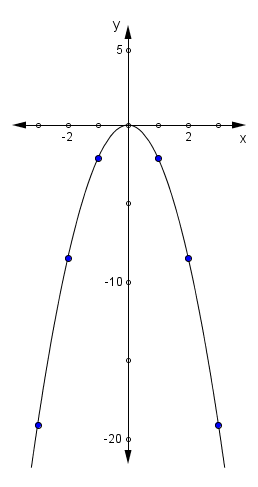### Home > MC2 > Chapter 11 > Lesson 11.3.1 > Problem11-92

11-92.

Make a table for the rule $y = -2x^{2}$ that includes $x$-values from $-3$ to $3$. Graph the rule on graph paper.

Substitute values from $−3$ to $3$ into $x$ to solve for the $y$-values.

Fill in the table.

Graph the rules based on the points from the table.

Shown to the right.

x

y

$-3$

$-2$

$-1$

$0$

$1$

$2$

$3$